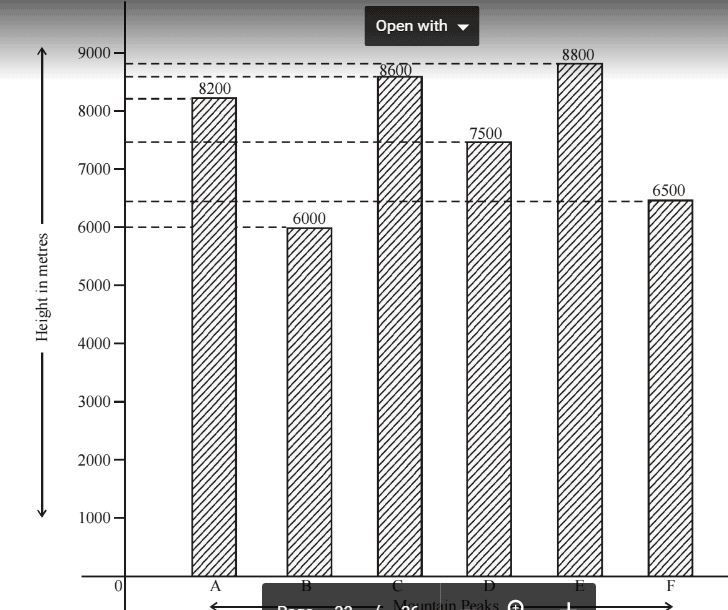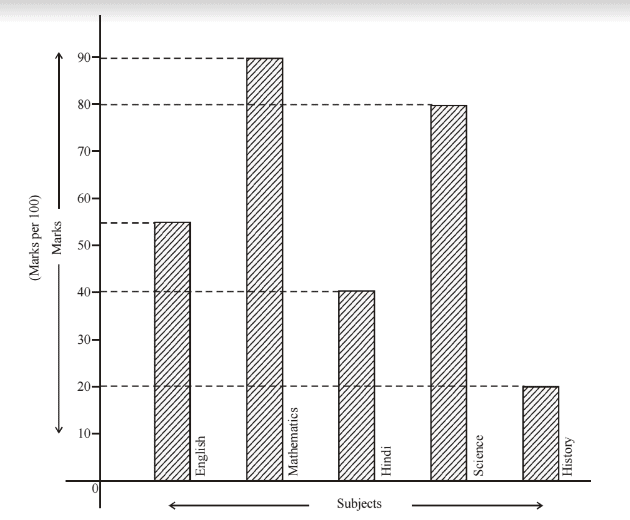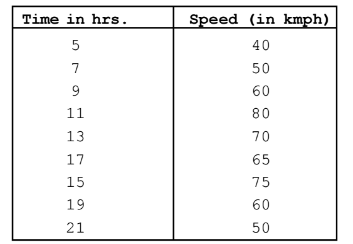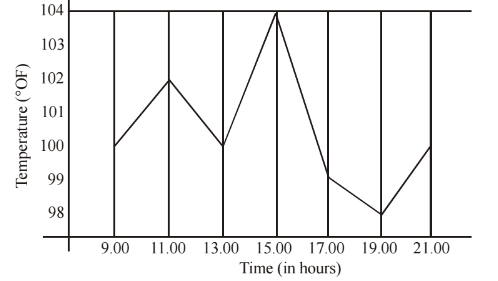Courses

# Statistics - Olympiad Level MCQ, Class 9 Mathematics

## 30 Questions MCQ Test Olympiad Preparation for Class 9 | Statistics - Olympiad Level MCQ, Class 9 Mathematics

Description
This mock test of Statistics - Olympiad Level MCQ, Class 9 Mathematics for Class 9 helps you for every Class 9 entrance exam. This contains 30 Multiple Choice Questions for Class 9 Statistics - Olympiad Level MCQ, Class 9 Mathematics (mcq) to study with solutions a complete question bank. The solved questions answers in this Statistics - Olympiad Level MCQ, Class 9 Mathematics quiz give you a good mix of easy questions and tough questions. Class 9 students definitely take this Statistics - Olympiad Level MCQ, Class 9 Mathematics exercise for a better result in the exam. You can find other Statistics - Olympiad Level MCQ, Class 9 Mathematics extra questions, long questions & short questions for Class 9 on EduRev as well by searching above.
QUESTION: 1

Solution:
QUESTION: 2

###Write the ratio of the heights of highest peak and the lowest peak :-

Solution: Highest peak =8800m Lowest peak=6000m Ratio=highest peak/lowest peak =8800/6000 =22/15 =22:15(Ans).
QUESTION: 3

###Which peak is second highest ?

Solution:
QUESTION: 4When the heights of the given peaks are written in ascending order, then what is the average of the middle

two peaks ?

Solution:
QUESTION: 5At what subject is the student sharp ?

Solution:
QUESTION: 6In which subject is the student poor ?

Solution:
QUESTION: 7What are the average marks obtained by the student ?

Solution:
QUESTION: 8What is the percentage obtained by the student ?

Solution:
QUESTION: 9What is the ratio of the highest marks to the lowest marks obtained by the student ?

Solution:
QUESTION: 10What is the percentage increase in speed during 9.00 hrs and 11.00 hrs ?

Solution:
we have to find the increase in speed in terms of percentage.so increase in speed between 9 to 11 hrs is (80 - 60)kmph
that is 20kmph
so increase in percentage =difference between two no / original no. *100
=20kmph/60kmph*100=100/3%
QUESTION: 11What is the percentage of decrease in speed during 19.00 hrs and 21.00 hrs ?

Solution:
QUESTION: 12What is the average speed between 5.00, 7.00 and 9.00 hrs ?

Solution:
QUESTION: 13What is the percentage decrease is temperature between 17.00 hrs and 19.00 hrs ?

Solution:
QUESTION: 14

The arithmetic mean of first five natural number is :-

Solution:
QUESTION: 15

If the arithmetic mean of 6, 8, 5, 7, x and 4 is 7, then x is :-

Solution:
QUESTION: 16

The arithmetic mean of first ten natural numbers is :-

Solution:
QUESTION: 17

The weight (in kg) of 5 men are 62, 65, 69, 66 and 61. The median is :-

Solution:
QUESTION: 18

If in a data, 10 numbers arranged in increasing order. If the 7th entry is increased by 4, then the median increase by :-

Solution:
QUESTION: 19

The mean of x, x + 3, x + 6, x + 9 and x + 12 is :-

Solution:
QUESTION: 20

The daily sale of kerosene (in litres) in a ration shop for six days is as follows : 75, 120, 12, 50, 70.5 and

140.5 The average daily sale is :-

Solution:
QUESTION: 21

The mean of five numbers is 27. If one of the numbers is excluded the mean gets reduced by 2. The excluded

number is :-

Solution:
QUESTION: 22

The median of the following data 46, 64, 87, 41, 58, 77, 35, 90, 55, 33, 92 is :-

Solution:
QUESTION: 23

The mean of 10 observation is 25. If one observation namely 25, is deleted, the new mean is :-

Solution:
QUESTION: 24

The mean of 10 observation is 25. If one observation namely 25, is deleted, the new mean is :-

Solution:
QUESTION: 25

The daily earnings (in rupees) of 10 workers in a factory are 8, 16, 19, 8, 16, 19, 16, 8, 19, 16. The median

wage is :-

Solution:
QUESTION: 26

The mean of 6, y, 7, x and 14 is 8. Then :-

Solution:
QUESTION: 27

Find the mean of 994,996,998,1002 and 1000.

Solution: 994+996+998+1002+1000=4990then 4990/5 =998
QUESTION: 28

The runs scored by Sachin in 5 test matches are 140, 153, 148, 150 and 154 respectively. Find his mean:-

Solution:
QUESTION: 29

The mean of first six multiples of 4 is :-

Solution:
QUESTION: 30

The mean of first five prime numbers is :-

Solution: